Refer to our Texas Go Math Grade 6 Answer Key Pdf to score good marks in the exams. Test yourself by practicing the problems from Texas Go Math Grade 6 Lesson 13.2 Answer Key Addition and Subtraction Inequalities.

Modeling One-Step Inequalities

You can use algebra tiles to model an inequality involving addition.

On a day in January in Watertown, NY, the temperature was 5 °F at dawn. By noon it was at least 8 °F. By how many degrees did the temperature increase?A. Let x represent the increase in temperature. Write an inequality.B. The model shows 5 + x ≥ 8. How many tiles must you remove from each side to isolate x on one side of the inequality? :
Circle these tiles. _________C. What values of x make this inequality true? Graph the solution of the inequality on the number line.
x ≥ ____________Reflect

Question 1.
Analyze Relationships How is solving the inequality 5 + x ≥ 8 like solving the equation 5 + x = 8? How is it different?
Solving the equation, we get exactly one solution, but solving the inequality, we get usually more values than one which are solutions.

Solve each inequality. Graph and check the solution.

Question 2.
y – 5 ≥ -7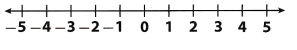We will, add 5 to both sides and get:
y ≥ 2
Now, we will graph the solution.Next thing we will do is to check the solution.
We will substitute a solution from the shaded part of our number line into the original inequality.
So, we will substitute 0 for y into y – 5 ≥ -7 and get:
0 – 5 ≥ 7
5 ≥ -7
So, the inequality is true.

Question 3.
21 > 12 + xWe will subtract 12 from both sides and get:
9 > x
Now, we will graph the solution:Next thing we will do is to check the soLution.
So, we will substitute 3 for x into 21 > 12 + x:
21 > 12 + 3
21 > 15
So, the inequality is true

Reflect

Question 4.
If you were to graph the solution, would all points on the graph make sense for the situation?
Not all, the points on the graph make sense for the situation, for example, in previous example, Junes dog will never weighs 1 pound, but – 1 is a solution of inequality from that task.

Question 5.
Write a real-world problem that can be modeled by x – 13> 20. Solve your problem and tell what values make sense for the situation.
Today temperature decreased by 13°C compared to yesterday’s temperature, but it is not greater than 20°.
For example, this situation can be described using the model in the task and its solution is x > 26
But, values greater than 40°C do not make sense for this situation.

Question 1.
Write the inequality shown on the model. Circle the tiles you would remove from each side and give the solution. (Explore Activity)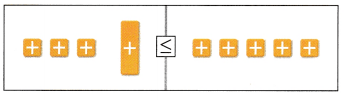Inequality: ______________
Solution: ______________

Solve each inequality. Graph and check the solution. (Example 1)

Question 2.
x + 4 ≥ 9 ______________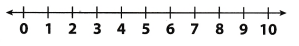First, we will subtract 4 from both sides and get:
x ≥ 5
Now, we will graph the solution:Now we will check our solution substituting a solution from the shaded part of our number line into the original inequality.
We will substitute 6 for x into x + 4 ≥ 9 and get:
6 + 4 ≥ 9
10 ≥ 9
So, the inequality is true

Question 3.
5 > z – 3 ______________We will add 3 to both sides and get:
8 > z
Now, we will graph the solution:Now we will check our solution substituting a solution from the shaded part of our number line into the original inequality.
We will substitute 2 for z into 5 > z – 3 and get:
5 > 2 -3
5 > -1
So, the inequality is true.
z < 8

Question 4.
t + 5 > 12 ______________We will subtract 5 from both sides and get:
t > 7
Now, we will graph the solution.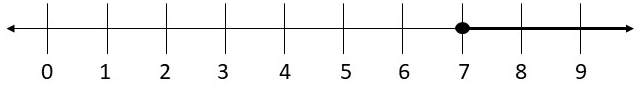Now we will check our solution substituting a soLution from the shaded part of our number line into the original inequality.
We will substitute 10 for t into t + 5 > 12 and get:
10 + 5 > 12
15 > 12
So, the inequality is true.
t > 7

Question 5.
y – 4 < 2 ______________We will add 4 to both sides and get:
y < 6
Now, we will graph the solution:Now we will check our solution substituting a solution from the shaded part of our number line into the original inequality.
We will substitute 3 for y into y – 4 < 2 and get:
3 – 4 < 2
-1 < 2
So, the inequality is true.
y < 6

Question 6.
Write a real-world problem that can be represented by the inequality y – 4 < 2. Solve the inequality and tell whether all values in the solution make sense for the situation. (Example 2)
Sara has appLes and when she gave 4 of them to her sister, she has left less than 2.
How much apples Sara could have?
We will use Addition Property of Inequality, we will add 4 to both sides and get:
y < 6 So, Sara has less than 6 apples, but this a real-world situation makes sence only for the following values of y : 5.4, where y represent number of apples Sara had.

Essential Question Check-In

Question 7.
Explain how to solve 7 + x ≥ 12. Tell what property of inequality you would use.
We will use the Subtraction Property of Inequality in order to solve this inequality, subtracting 7 from both sides and get: x ≥ 5

Solve each inequality. Graph and check the solution.

Question 8.
x – 35 > 15 _______________We will, use Addition Property of inequality, we will add 35 to both sides and get:
x > 50
Now, we will graph the solution.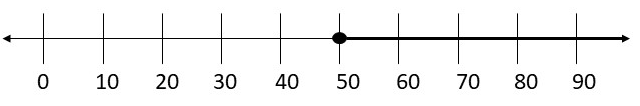Now we will check our solution substituting a solution from the shaded part of our number line into the original inequality.
We will substitute 60 for x into x – 35 > 15 and get:
60 – 35 >15
25 > 15
So, the inequality is true.
x > 50

Question 9.
193 + y ≥ 201 _______________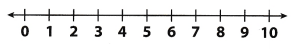We will use Subtraction Property of Inequality in order to solve this inequality, we will subtract 193 from both sides and get:
y ≥ 8
Now, we will graph the solution.Now we will check our solution substituting a solution from the shaded part of our number line into the original inequality.
We will, substitute 10 for y into 193 + y ≥ 201 and get:
193 + 10 ≥ 201
203 ≥ 201
So, the inequality is true
y ≥ 8

Question 10.
y – 5 ≥ -15 ________________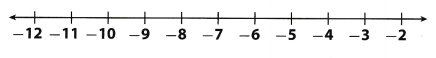We will use Addition Property of Inequality, we will add 5 to both sides and get:
y ≥ -10
Now, we will graph the solution.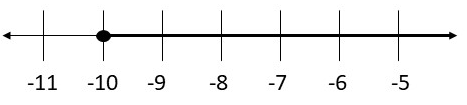Now we will check our solution substituting a solution from the shaded part of our number line into the original inequality.
We will substitute -8 for y into y – 5 ≥ – 15 and get:
8 – 5 ≥ -15
12 ≥ -15
So, the inequality is true.
y ≥ -10

Question 11.
15 ≥ z + 26 ________________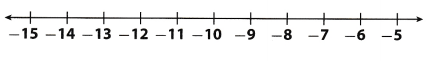We will first use first Subtraction Property of Inequality.
We will subtract 26 from both sides and get:
-11 ≥ z
Now, we will graph the solution:Now we will check our solution substituting a solution from the shaded part of our number line into the original inequality.
We will substitute 15 for z into 15 ≥ z + 26 and get:
15 ≥ -15 + 26
15 ≥ 11
The inequality is true
z ≤ -11

Write an inequality to solve each problem.

Question 12.
The water level in the aquarium’s shark tank is always greater than 25 feet. If the water level decreased by 6 feet during cleaning, what was the water level before the cleaners took out any water?
Let x be the water level before the cleaners book out any water. We have the following inequality according to information given in task:
x – 6 > 25
We will use Addition Property of Inequality adding 6 to both sides in order to solve this inequation and get:
x > 31
So, before the cleaners took water, the water level was greater than 31.

Question 13.
Danny has at least $15 more than his big brother. Danny’s big brother has$72. How much money does Danny have?
Let x be sum of money Danny has. According to given informations in this task, we have the following inequality:
x – 72 ≥ 15
We will use Addition Property of Inequality adding 72 to both sides and get:
x ≥ 87
So, conclusion is that Danny has at least $87. Question 14. The vet says that Ray’s puppy will grow to be at most 28 inches tall. Ray’s puppy is currently 1 foot tall. How much more will the puppy grow? Answer: First, we will convert 1 foot to inches, it is 12 inches. Let x be how much more will, the puppy grow. According to informations given in this task, we will have the following inequality: 12 – x ≤ 28 We will use Subtraction Property of Inequality and subtract 12 from both sides and get: x ≤ 16 So, the puppy will grow for 16 inches at most. Question 15. Pierre’s parents ordered some pizzas for a party. 4.5 pizzas were eaten at the party. There were at least 5$$\frac{1}{2}$$ whole pizzas left over. How many pizzas did Pierre’s parents order? Answer: Let x be number of pizzas Pierres parents ordered. According to informations given in this task, we will have the following inequality: x – 4.5 ≥ 5$$\frac{1}{2}$$ We will first convert decimals into fraction: 5$$\frac{1}{2}$$ = $$\frac{11}{2}$$ Now, we will use Adding Property of Inequality, we will add $$\frac{9}{2}$$ to both sides and get: x ≥ $$\frac{11}{2}$$ + $$\frac{9}{2}$$ x ≥ $$\frac{20}{2}$$ x ≥ 10 So, Pierre’s parents ordered at least 10 pizza’s. Question 16. To get a free meal at his favorite restaurant, Tom needs to spend$50 or more at the restaurant. He has already spent $30.25. How much more does Tom need to spent to get his free meal? Answer: Let x be sum of money Tom needs to spent to get his free meal. According to informations given in this task, we will have the following inequality: 30.25 + x ≥ 50 In order to solve this inequality, we will use Subtraction Property of Inequality, we will subtract 30.25 from both sides and get: x ≥ 19.75 So, Tom needs to spend$19.75 or more to get his free meal.

Question 17.
Multistep The table shows Marco’s checking account activity for the first week of June.a. Marco wants his total deposits for the month of June to exceed $1,500. Write and solve an inequality to find how much more he needs to deposit to meet this goal. Answer: Let x be deposit needed to Marco which he add to$520.45 to exceed $1,500. Using informations in t his task, we have the following inequality. 520.45 + x > 1,500 We will use Subtraction Property of Inequalities and subtract 520.15 from both sides and get: x > 979.55 So, Marco will need more than$979.55 to exceed $1,500. b. Marco wants his total purchases for the month to be less Write and solve an inequality to find how much more he and still meet this goal. Answer: Marco’s total purchases for the first week of June is: 16.50 + 24.00 + 22.82 = 93.32 Let t be the sum of money Marco can spend over$ 93.32 in order to his total purchase be less than $450. According to all informations given in task, we have the following: 93.32 + x < 450 We will use Subtracting Property of inequalities and subtract 93.32 from both sides and get: x < 356.68 So, his purchases need to he less than$ 356.68 to meet his goal.

c. There are three weeks left in June. If Marco spends the same amount in each of these weeks that he spent during the first week, will he meet his goal of spending less than $450 for the entire month? Justify your answer. Answer: Marco spent 93.32 in first week of June. We will multiply this result by 3 because there are 3 more weeks left in June and get: 3 ∙ 93.32 = 279.96 We got that for whole month he will spend$ 279.96 which is less that \$450. so. he will meet his goal.

H.O.T. Focus on Higher Order Thinking

Question 18.
Critique Reasoning Kim solved y – 8 ≤ 10 and got y ≤ 2. What might Kim have done wrong?
She did not use proper Property of Inequalities. Here, instead of Adding Property, she used Subtracting Property and got wrong result.
So, using Adding Property and add 8 to both sides, we get: y ≤ 18

Question 19.
Critical Thinking Jose solved the inequality 3 > x + 4 and got x < 1. Then, to check his solution, he substituted -2 into the original inequality to check his solution. Since his check worked, he believes that his answer is correct. Describe another check Jose could perform that will show his solution is not correct. Then explain how to solve the inequality.
For, example, if he substitute 0 for x into inequality, we will get: 3 > 0 + 4
3 > 4
So, we can notice that this inequality is incorrect.
So, the right way to solve this inequality is to use Subtracting Property of Inequalities and subtract 4 from both sides and get:
-1 > x
So, the solution is x < -1

Question 20.
Look for a Pattern Solve x + 1 > 10, x + 11 > 20, and x + 21 > 30. Describe a pattern. Then use the pattern to predict the solution of x + 9, 991 > 10,000.